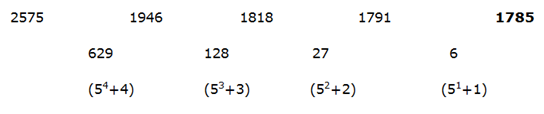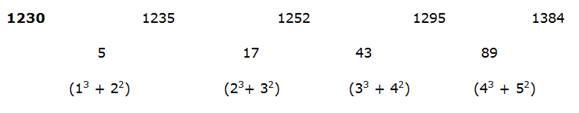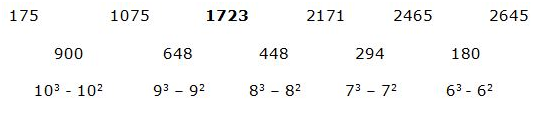# SBI Clerk Prelims 2021 Quantitative Aptitude Questions (Day-46)

Dear Aspirants, Our IBPS Guide team is providing new series of Quantitative Aptitude Questions for SBI Clerk Prelims 2020 so the aspirants can practice it on a daily basis. These questions are framed by our skilled experts after understanding your needs thoroughly. Aspirants can practice these new series questions daily to familiarize with the exact exam pattern and make your preparation effective.

Start Quiz

Missing number series

Directions (01-05): What value should come in the place of (?) in the following number series?

1) 2575, 1946, 1818, 1791, ?

A.1789

B.1889

C.1783

D.1683

E.1785

2) 200, ?, 167.5, 438.75, 1575.625

A.105

B.205

C.55

D.305

E.155

3) ?, 1235, 1252, 1295, 1384

A.1235

B.1230

C.1225

D.1220

E.1233

4) 175, 1075, ?, 2171, 2465, 2645

A.1733

B.1728

C.1722

D.1700

E.1723

5) 73, ?, 120, 188, 313

A.80

B.85

C.87

D.83

E.82

Simplification

Directions (06-07): What value should come in the place of (?) in the following questions?

6) (943 ÷ 23) * (18/41) * ? = 612

A.31

B.34

C.37

D.39

E.36

7) √4761 – √3249 + √6561 =?

A.-49

B.59

C.93

D.-79

E.89

SI AND CI

8) A invests Rs.4800 at simple interest scheme at x % per annum for 4 years and after 4 years he received the total amount is Rs.7680. If B invests the same amount at simple interest scheme at (x + 3)% per annum for 5 years, find the interest amount received by B?

A.Rs.2340

B.Rs.5430

C.Rs.4320

D.Rs.2880

E.None of these

Partnership

9) A started the business with the investment of Rs.4500 and after 4 months B joined with the business. At the end of the year, the total profit is Rs.17000 and A’s profit share is Rs.9000, what is B’s investment?

A.Rs.4000

B.Rs.5000

C.Rs.6000

D.Rs.8000

E.None of these

Profit and loss

10) If the difference between the marked price and cost price of the article is Rs.180 and the shopkeeper offers 10% discount while he gets 20% of the profit, what is the marked price of the article?

A.Rs.720

B.Rs.780

C.Rs.800

D.Rs.740

E.Rs.760200 * 0.5 + 5 = 105

105 * 1.5 + 10 = 167.5

167.5 * 2.5 + 20 = 438.75

438.75 * 3.5 + 40 = 1575.62573 + (3+ 2+ 13) = 87

87 + (4+ 3+ 23) = 120

120 + (5+ 4+ 33) = 188

188 + (6+ 5+ 43) = 313

(943 ÷ 23) * (18/41) * ? = 612

(41 * 18)/(41 * ?) = 612

? = 34

√4761 – √3249 + √6561 =?

69 – 57 + 81 =?

? = 93

SI = (P * N * R)/100

7680 – 4800 = (4800 * x * 4)/100

x = 15%

SI received by B = (4800 * (15 + 3) * 5)/100

= 4320

Profit ratio of A and B = (4500 * 12): (x * 8)

= 6750: x

6750/(x + 6750) = 9000/17000

9x + 6750 * 9 = 17 * 6750

9x = 54000

x = 6000

MP – CP = 180

CP = MP – 180

MP * 90/100 = (MP – 180) * 120/100

3MP = 4MP – 720

MP = 720

 Check Here to View SBI Clerk Prelims 2021 Quantitative Aptitude Questions Day – 45 Day – 44 Day – 43